beginning of content:

Many students who come into my AP Calculus class place far too much faith in calculator results, blindly accepting results without questioning whether they’re correct or even reasonable. Over the years, I’ve assembled examples whose main purpose is to develop in students a healthy skepticism for machine results. In a few cases, the examples also serve as a starting point for lively discussions about calculus concepts. Most of these examples can be adapted to any calculator model, with minor variations.

## Example 1: Round Off Error and Limited Precision

Whenever a finite precision machine attempts to add numbers of vastly different magnitudes, accuracy can be lost. Try this calculation:

(10^ 15 + 7.2 − 10^ 15) * 100

The result should be 720, not 0. The problem is that every digital calculator uses a finite number of digits to store numbers (thus all numbers that get stored are rational). The TI-83, for instance, keeps 13 digits. When 10^ 15+7.2 is calculated, therefore, the result is exactly 10^ 15 (the 7 and the 2 are past the 13th digit). Now, when 10^ 15 is subtracted, the result is 0. Note that this example can be used to illustrate that, to a calculator, addition is not associative. Repeat the example, but change the order to (10^ 15 −10^ 15 + 7.2)*100. Now, the result is correct since at no time does the machine need to add two numbers whose magnitudes are vastly different. The problem isn't the magnitude of the numbers involved in the calculation, but rather their relative magnitudes. When you try to add two numbers of vastly different magnitudes, a and b, you'll have problems.

Bizarre effects of round off errors can be illustrated graphically. Graph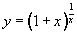in a window centered at about (0,2.7). Then repeatedly zoom in with a horizontal factor of 10 and a vertical factor of 1 (thus leaving the vertical scale unchanged). After about 12 or 15 zooms (depending on how many digits of precision your calculator carries), the graph goes haywire. The problem is that x is very small, and when it is added to 1 (in the expression 1 + x), the result is not exact. In fact, eventually (if you zoom in a couple more times), the result becomes exactly 1. The figure below left shows the graph on a TI-86 in the window x ∈ [-6.3E-13, 6.3E-13] and y ∈ [0,5.4], and below right in the window x ∈ [-6.3E-14, 6.3E-14] and y ∈ [0,5.4]. Shocking!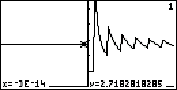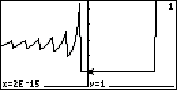## Example 2: Approximating a Derivative Numerically

When a calculator without a built-in Computer Algebra System (CAS) finds a numeric derivative, typically it uses an approximation like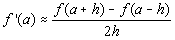This approximation is called the symmetric difference quotient.

[The function graphed here is y = x^ 3-x, on the interval -.9 to -.3. The tangent is graphed at a = -.6.]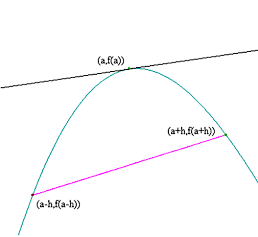Note that points on either side of a are sampled, and the slope of the line segment connecting them is returned as an approximation to the slope of the tangent line at a. This approximation is pretty good for most purposes, but can cause trouble for the unwary user. In particular, strange results occur at a place where f'(x) doesn't exist, or at a place where f(x) is not continuous. Try these:

• Calculate the numerical derivative of x^ (2/3) with respect to x at x = 0.
• Calculate the numerical derivative of arctan(1/x) with respect to x at x = 0.

In the first example, you get a result of 0, even though the function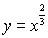is not differentiable at x = 0. The problem is that the value of x^ (2/3) is the same at x = -a and x = a. The machine does not detect the cusp in the graph at x = 0. In fact, the symmetric difference quotient applied to any even function at x = 0 will give 0 as the result.

In the second example, the result is a large positive number, even though the function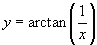is not even continuous at x = 0. If you graph y = arctan(1/x) centered at the origin, you can see what's going on. The machine calculates and returns the slope of the secant line connecting points on either side of the discontinuity at x = 0. This secant line is very steep indeed!

## Example 3: Approximating an Integral Numerically

Here's an example where the calculator's built-in numerical integration algorithm can be fooled. (CAS-equipped machines do better on this one!)

Try to evaluate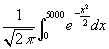The result should be 0.5, not 0. The problem is that the numerical integration algorithm stops taking larger partitions as soon as a few successive approximations agree. The first few approximations give a sum of zero, since the integrand is very close to zero for large values of x. Try this same integral with an upper limit of 500 instead of 5000 and you’ll get a better result.

## Example 4: Numeric Solver

The root finding algorithm built into the TI-8x will occasionally fail to find even simple roots. Try these:

Find a root of y = x2 using your calculator's numeric solver. (MATH 0 on the TI-82/3)

Find a point of intersection of the graphs of Y = sin(X)/X and Y = 1/X. Notice that the graphs never cross, though they intersect an infinite number of times (whenever sin(X) = 1). The intersect feature simply relies on the numeric solver applied to the difference between the selected functions. If that difference doesn’t change sign, the solver is prone to missing the intersection point.

The problem in both cases is that the root-finding algorithm is overly dependent at the outset on finding a sign change for the function. Whenever the graph of a function is tangent to the x-axis, there is potential for the numeric solver to fail to find the root.

## Example 5: Hidden Graphic Behavior

Any machine grapher is forced to sample only a discrete subset of inputs to a function in whatever window is specified. Consequently, the graphs produced can miss "hidden" behavior. Try graphing these functions

• Y1=sin(47X)
• Y2=sin(48X)
• Y3=sin(49X)

in the zoom trig window on any TI calculator. (For other calculators, you may have to adjust the coefficients to see the effect, depending on screen resolution and the settings of the trig window.) Many calculators will set the distance between columns to π/24 in the trig window, so that tracing on the graph hits all the standard angles. The value of π/24 is approximately .1309. The periods of our functions are 2π/47 ≈ .1337, 2π/48 ≈ .1309, and 2π/49 ≈ .1282. When a graph is drawn, the calculator simply samples values of x across the graph screen, selecting one x in each column of pixels. For example, suppose the machine evaluates our functions at x = 0. The next x sampled is x = .1309, and our functions are evaluated there. The machine connects the points by a line. But as x increased from 0 to .1309, Y1 has nearly completed a full period, Y2 has completed exactly one full period, and Y3 has completed a bit more than one period. The grapher has missed almost all of that behavior! See the illustration below: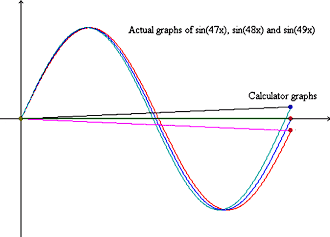It is relatively easy to crank out other “hidden behavior” examples by starting with maximum and minimum points that are close together horizontally. This example comes from starting with a derivative that has sign changes at x = 0 and x = 1/18. Graph y = 12x3 − x2 + 1 in a zoom decimal window. It appears that the function is always increasing, but if you look in a window with x ∈ [-.05, .1] and y ∈ [.998, 1.002], you see the previously hidden extrema.

## Example 6: A CAS Error

Students may believe that machines equipped with a Computer Algebra System are immune from errors. This is not so. Here's a genuine CAS error that occurs with the TI-89 limit command:

Try limit(sin(x+1/x)-sin(x),x, ∞). The TI-89 returns undefined. The correct limit is 0. The TI-89 doesn't expand the trig function when evaluating limits. If you repeat the example this way: limit(tExpand(sin(x+1/x))-sin(x),x, ∞), you'll get the correct result.

## Authored by

• Mark Howell
Gonzaga College High School
Washington, District of Columbia